Home PHYSICS TOPIC 4: OPTICAL INSTRUMENT | PHYSICS FORM 3

# TOPIC 4: OPTICAL INSTRUMENT | PHYSICS FORM 3

1622
183## TOPIC 4: OPTICAL INSTRUMENT | PHYSICS FORM 3

Optical instruments are devices which are used to help the human eye views small or distant objects more clearly. ​​

They use a combination of lenses (mirrors) to produce an enhanced image of an object.​​

#### Optical instruments consist of:​​

• Simple Microscope ​​
• Compound Microscope ​​
• Astronomical Telescopes ​​
• Simple lens Camera ​​
• Projection lantern ​​
##### ​​Simple Microscope ​​It consists of a biconvex lens which may be hand-held or placed in a simple frame. It is sometimes referred to as a​​ magnifying glass.  ​​​​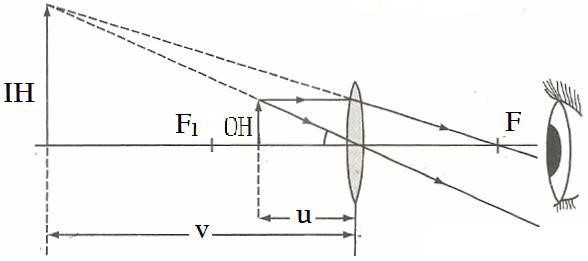​​

Object is placed between​​ f and lens​​ ( u < f )​​

Virtual, upright and magnified image of the object is formed. ​​

The image appears clearest when it is about 25 cm from the eye, V = 25cm. This distance is called the​​ near point​​ (D). ​​

The nearer the object is to the lens, the further and larger the image formed. ​​

• Magnification​​ ​​
• Consider the diagram below ​​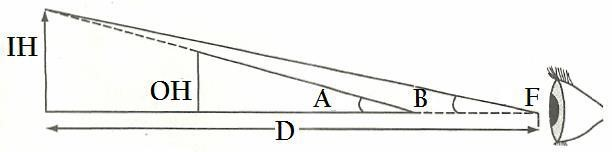​​Where ​​
• = angle subtended by the object ​​
• = angle subtended by the virtual image ​​
• D = distance between F and image ​​
• Lateral Magnification​​

The lateral magnification of a simple lens is the ratio of the image height (IH) to the object height (OH).​​

𝑴= 𝐇𝐈 𝐇𝐎

Linear Magnification​​ ​​

Is also given by the ratio of the image distance​​ v​​ to the object distance​​ u. ​​

​​ ​​​𝑴 ​​= 𝑽𝑼

Angular Magnification (Magnifying power)​​

Is the ratio of the angle subtended at the eye by the object when viewed through the magnifying glass (B) to the angle subtended at the eye by the object when viewed with naked eyes (A) ​​

​​​𝒎 = 𝑩 𝑨

From the diagram above ​​

Tan B =​​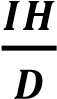Since:  ​​ ​​ ​​​​ angle​​ B​​ is small, it can be expressed as  ​​ ​​​​ B = IH/D​​ ​​

If the angle B subtended by the virtual image. Ignoring the small distance between the eye and the magnifying lens ​​​​Then: Tan A = OH/D​​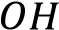Since: angle B is small, it can be expressed as  ​​ ​​ ​​​​ A = ​​ …………… 2 ​​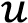​​ Substitute equation 1 and 2 in  ​​ ​​​​ m = B/A​​ ​​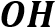𝑴= 𝑼 𝑶𝑯 𝑽

𝑴 ​​= 𝑽𝑼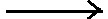𝑀 = (𝑂𝐻) 𝑥 (​​ 𝑉​​ )​​ ​​ 𝑈 𝑂𝐻

When the image is at the near point, v = 25 cm. Therefore, using the lens formula, ​​

•​​ ​​​​ … ​​ (make u the subject) ​​​​ ​​​​substitute into​​ M = V/U ​​​​

M = v/(25f/(25+f)) ​​

Therefore:  ​​ ​​ ​​ ​​ ​​ ​​ ​​​​

𝑴 ​​= (𝟐𝟓𝒇) + 𝟏

#### ​​ Individual task – 5:1​​

1. A simple microscope with a focal length of 5 cm is used to read division of scale 1.5 mm in size. How large will the size of the divisions as seen through the simple microscope be? ​​ (ANS: 9 mm when viewed through the simple microscope.)​​

##### Uses​​ ​​
• It is used to view specimen in the laboratory ​​
• It is used to read small print ​​
• ​​ Compound Microscope​​ ​​
• A Compound microscope is composed of two convex lenses of short focal lengths placed in a tube.​​
• The two lenses are separated by a certain fixed distance. ​​
• The lens near the object is called the​​ Objective Lens​​ while the lens near the eye is called the​​ Eyepiece Lens​​

See the figure below​​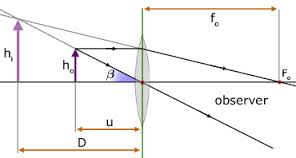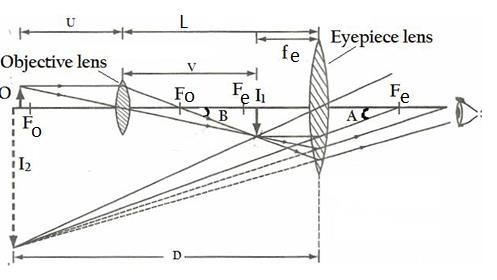​​ Mode of Action​​ ​​

Objective lens enlarges object to form image,​​ I1​​ ​​

Eyepiece lens enlarges​​ I1​​ (As object) to form image​​ I2.​​ The image produced is magnified, virtual and inverted compared to the original object ​​

In order​​ I2​​ to be seen clearly, the eyepiece lens should be adjusted until​​ I2​​ ​​ is at the near point ,D​​ , from the eye as shown from the figure above​​

​​Magnification produced by a compound microscope​​

​​ Where​​ V​​ is the distance of​​ I1​​ and​​ U​​ is the distance of object(O) from the objective lens​​

But:​​​​………(i)​​

Also, the magnification produced by the eyepiece lens is given by,​​………… (ii)​​

Where​​ D​​ is the distance of​​ I2​​ from the eyepiece ​​

The total magnification (m) produced by a compound microscope​​ is the product of the magnification produced by the objective and the magnification produced by the eyepiece. (from equation i and ii), i.e​​

𝒎 = 𝒎𝒐

​​ 𝒙​​𝒎𝒆= 𝒎𝒐

𝒎𝒆 =

(𝒗𝒇𝒐𝟏)(𝑫𝒇𝒆−𝟏)

​​ Example ​​

1. A compound microscope has an objective lens of focal length 2 cm and eye piece of focal length of 6 cm. An object is placed 2.4 cm from the objective lens. If the distance between the objective lens and the eyepiece lens is 17 cm find:- (a) The distance of the final image from the eyepiece.​​

(b) The linear magnification.​​

Soln:​​

Given: ​​ fO​​ = 2 cm, fE​​ = 6 cm, uo​​ = 2.4 cm, L = 17 cm (a) Consider the figure below:​​ ​​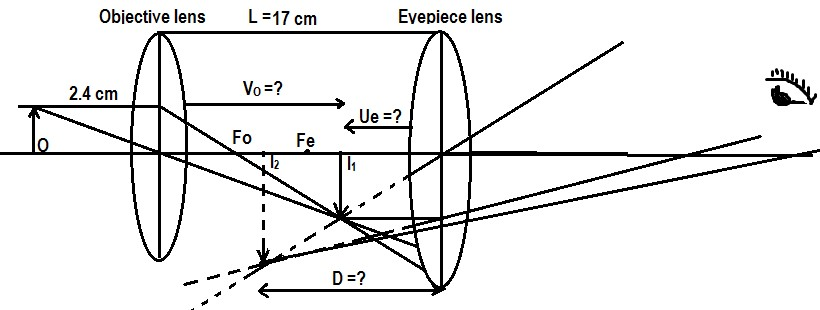​​

• ​​ Refraction from the objective lens:​​
• ​​ From:​​
• ​​ VO​​ = 12 cm, but VO​​ + UE​​ = L  ​​ ​​​​ →​​ Ue​​ ​​ = L – VO​​ = 17 cm – 12 cm = 5 cm​​
• ​​ Also consider the refraction from the eyepiece lens​​
• From:  ​​​​​​
• ​​ D = -30 cm, (According to real – is – positive the final image is virtual)​​
• ​​ ​​ The distance of the final image from the eyepiece is 30 cm ​​
• ​​ (b)​​ Linear magnification (total magnification)​​
• From:​​​​ ​​ ​​ ​​ ​​ ​​ ​​ ​​ ​​ ​​ ​​ ​​ ​​​​​​
• ​​ ​​ ∴ 𝒍𝒊𝒏𝒆𝒂𝒓 𝒎𝒂𝒈𝒏𝒊𝒇𝒊𝒄𝒂𝒕𝒊𝒐𝒏 𝒊𝒔 𝟐𝟎​​

#### ​​ Individual Task – 5:2​​

A compound microscope consists of two lenses of focal length 12 cm and 6 cm for the objective lens and the eyepiece lens, respectively. The two lenses are separated by a distance of 30 cm. The microscope is focused so that the image is formed at infinity. Determine the position of the object.  ​​ ​​​​ ANS:  ​​​​ U = 24 cm​​

A parallel beam of light falls on a converging lens arranged so that the axis lies along the direction of the light which is brought to focus 25 cm from the lens.​​ The​​ light then passes through a second converging lens of focal length 7.5 cm placed at 30 cm from the first lens. Calculate the position of the final image. Draw a ray diagram to show the final image formed.(ANS: D ​​ = 15 cm)​​

The total magnification produced by a compound microscope is 20. The magnification produced by the eye piece alone is 5. The microscope is focused on a certain object. The distance between the objective lens and the eyepiece is observed to be 14 cm. If least distance of distinct vision is 20 cm, calculate the focal lengths of the objective and eyepiece lenses.​​ ​​ ​​

##### Uses​​ ​​
• Observing Brownian motion in science ​​
• Studying microorganisms and cells in biology ​​
• Checking for infections caused by microorganisms in hospitals​​
• ​​ Astronomical Telescope​​ ​​
• It uses two convex lenses that is the objective lens and the eyepiece lens. ​​
• The objective lens has a large focal length while the eyepiece lens has a much shorter focal length as you compare to compound microscope ​​

​​ Mode of action​​ ​​

​The objective lens forms a real, inverted and diminished image of a distant object at its focal point,​​ fo. ​​​​

This becomes the object for the eyepiece lens.​​

​​The position of the eyepiece lens is adjusted until the object is at its focal point,​​ fe. ​​ ​​ This adjustment makes the final image to be formed at infinity.​​ ​​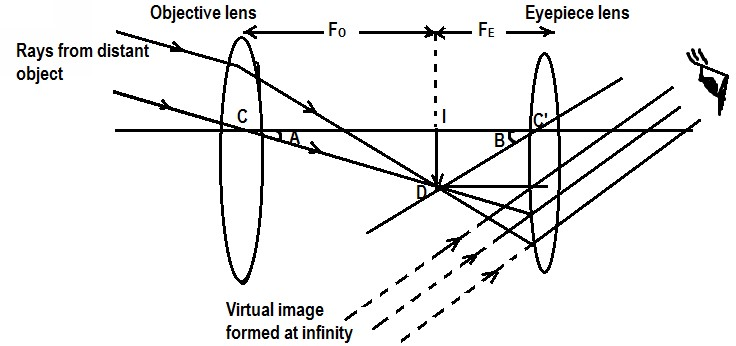​​​​ NB:​​ ​​

The final image obtained in the astronomical telescope is small compared to the original object. ​​

The image looks larger because it is very much closer to the observer’s eye. ​​

• Magnification produced by astronomical telescope​​

From: Angular magnification of the telescope​​ ​​ ​​ ​​ ​​ ​​​​Since both the object and the final image are at infinity, the​​ angles they subtend at the eye are the same as those they subtend at the objective and at the eyepiece lens, respectively. ​​

Assuming that: angle A and B are very small, then​​ tan A = A and tan B = B​​

Hence  ​​​​Since:​​ ​​​​ ……………… substitute​​ A​​ and​​ B​​ above​​  ​​ ​​ ​​ ​​ ​​ ​​ ​​​​Therefore the magnification produced by an astronomical telescope is the ratio of the focal length of the objective lens to that of the eyepiece lens, i.e. ​​

𝒎 =𝒇𝒐 𝒇𝒆 ​​ ​​ ​​ ​​ ​​ ​​ ​​ ​​ ​​​​

Example 1:​​

A refracting telescope has an objective lens with a focal length of 5.0 cm and an ​​ eyepiece with a short focal length of 0.02 m; calculate the magnifying power of such a telescope in its normal adjustment​​ Soln: ​​

Given: fo​​ = 5.0 cm, fe​​ = 0.02 m​​

From: ​​ ​​ ​​​​​​ Therefore the magnifying power is 250​​

What is the angular magnification of a telescope that has a 100 cm focal length objective and a 2.5 cm focal length eyepiece?​​ (ANS: 40)​​

Find the distance between the objective and eyepiece lenses in the telescope in the above problem needed to produce a final image very far (at infinity) from the observer, (ANS: 102.5 cm)​​

A large reflecting telescope has an objective mirror with a 10.0 m radius of curvature. What angular magnification does it produce when a 3.0 m focal length eyepiece is used?​​

A small telescope has a concave mirror with a 2.0 m radius of curvature for its objective. Its eyepiece is a 4.0 cm focal length. What is the telescope’s angular magnification? What angle is subtended by a 25,000 km diameter sunspot?​​

A 7.5x binocular produces an angular magnification of 7.5, acting like a telescope. If the binoculars have objective lenses with a 75.0 cm focal length, what is the focal length of the eyepiece lenses? ​​ ​​

##### Uses​​ ​​

An astronomical telescope is used to view distant objects like stars and other objects in space.​​

They are used in military bases to see enemies​​

Differences between Compound microscope and Astronomical telescope​​

##### Astronomical telescope​​

Objective lens has a smaller focal length than the eyepiece lens​​

Objective lens has a larger focal length than the eyepiece lens​​

Distance between the objective lens and the eye piece lens is greater than ​​ fo + fe​​

Distance between the objective lens and eye piece lens is equal to  ​​ ​​ ​​ ​​ ​​​​ fo + fe​​

It is used to see very small objects​​

It is used to see distant astronomical objects​​

It uses artificial light ​​

It uses natural light at the focal point​​

It is very small in shape as compared to telescope​​

Very large in size as compared to microscope​​

​​ Projection Lantern​​ ​​

Projection lanterns are used to display a large image on a screen. ​​

One example, is the slide projector that is the optical inverse of a camera. ​​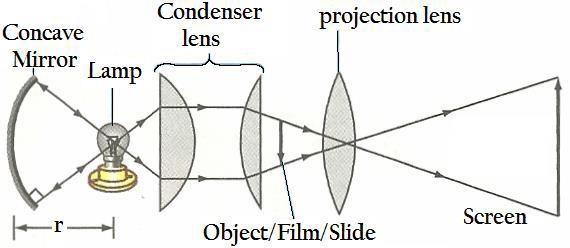​​Magnification​​ ​​

It is given by  ​​ ​​ ​​ ​​ ​​ ​​ ​​ ​​ ​​ ​​​​Example:​​

1. A projection lantern is used to give the image of a slide on a screen. If the image is 24 times as large as the slide and the screen is 72 m from the projecting lens, what is the position of the slide from the lens Soln:​​

Given: m = 24, v = 72 m, u =?​​

From:  ​​​​ ​​​​​​ ​​ ​ ​​ ​​ ​​ ​​ ​​ ​​ ​​​​∴ 𝑡ℎ𝑒 𝑠𝑙𝑖𝑑𝑒 𝑖𝑠 3𝑚 𝑓𝑟𝑜𝑚 𝑡ℎ𝑒 𝑙𝑒𝑛𝑠​​

2. A lantern projector using a slide of 2cm x 2cm projects a picture 1m x 1m onto a screen 12m from the projection lens. How far from the lens must the slide be?​​

Find the approximate focal length of the projection lens.​​

Soln:​​

Given: ​​ HO​​ = 2 cm, HI​​ = 1 m = 100 cm, v = 12 m =1200cm, ​​ u =?, ​​ f =?​​

From:​​​​Also:​​  ​​ ​​ ​​ ​​ ​​ ​​ ​​ ​​ ​​ ​​ ​​ ​​ ​​ ​​ ​​ ​​ ​​ ​​ ​​ ​​ ​​ ​​ ​​ ​​ ​​ ​​ ​​ ​​ ​​ ​​ ​​ ​​ ​​ ​​ ​​ ​​ ​​ ​​ ​​ ​​ ​​ ​​ ​​ ​​ ​​ ​​ ​​ ​​ ​​​​​​ ∴ 𝒕𝒉𝒆 𝒔𝒍𝒊𝒅𝒆 𝒎𝒖𝒔𝒕 𝒃𝒆 𝟐𝟒 𝒄𝒎​​ from the lens and the approximate focal length is 23.5 cm​​

1. A projection lantern is used to project a slide measuring 3 cm x 3 cm onto a screen 12 m from the projection lens. If the size of the screen is 1.5 m x 1.5 m, how far from the lens must the slide be for the image to fill the entire screen?  ​​ ​​ ​​ ​​ ​​ ​​ ​​ ​​ ​​ ​​ ​​ ​​ ​​ ​​ ​​ ​​ ​​ ​​​​ ANS: ​​ U = 24 cm.​​ ​​

##### Uses of Projection Lantern​​ ​​
• Projection of films, slides and transparencies. ​​
• Projection of opaque objects, i.e. episcopic projection. ​​
• In searchlights and headlights. ​​
• In physical experiments such as projection of the spectrum, polarisation experiments and interference experiments. ​​
• Projection of minute objects, i.e. the projection microscope. ​​
##### ​​ Lens Camera​​ ​​

The lens is the image-forming device on a camera. ​​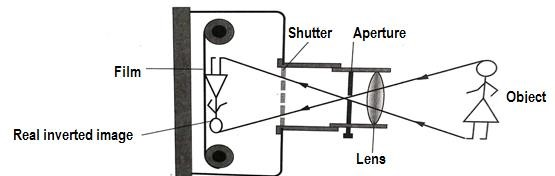Basic types of Lenses ​​

• Normal (standard lens) ​​
• wide angle lens ​​
• telephoto (long-focus lens) ​​

​​ Normal (standard) Lens​​ ​​

• The viewing is much wider-about 50 degrees. ​​
• The objects appear normal in size and shape, relative to the picture background​​

Wide Angle Lens​​ ​​

• The viewing is much wider-about 90 degrees. ​​
• Used to make smaller objects look larger or to photograph large objects from close up. ​​

Telephoto Lens​​ ​​

It has wider fields of view than normal lenses. ​​

They show an enlarged detail of the image over the same film area. ​​

##### ​​ Parts of the Lens Camera​​

Diaphragm​​

The diaphragm determines the amount of light that passes through the lens by changing the size of the aperture. ​​

NB: Aperture is an opening whose diameter is adjustable ​​

Shutter​​ ​​

The shutter is a mechanical device that acts as a gate, controlling the duration of time that light is allowed to pass through the lens and fall on the film. ​​

Viewfinder​​ ​​

The viewfinder defines the area covered by the lens that is in use on the camera. ​​

​​ Film​​ ​​

The film is a light-sensitive surface of the camera. ​​

Mode of Action ​​

The image of the object must be sharply focused on the film by adjusting the distance of the lens from the film ​​

After focusing and correctly setting the aperture size and shutter time ,the click button is pressed  ​​​​

The shutter opens to allow light to enter and expose the film to form an image of the object being photographed. The film is then developed to produce a photograph of the object​​

​​ Magnification​​ ​​​​

A lens camera of focal length 10 cm is used to take the picture of a girl 1.5 m tall. Determine the magnification of the image if the girl is 11m from the camera.(​​ m =0.009)​​ ​​

A lens camera has a lens of focal length 15 cm and a film (screen) of height 0.35 cm. How far would a boy of height 1.8 m stand from the camera so that his image just fits the film?​​

A professional photograph has a camera of focal length 2.5 cm. He uses it to take a photo of a tree of height 60 m. The distance between the lens of the camera and its film is 2.5 cm. Determine:​​ (a) Distance between the lens and the tree​​ (b) The height of the image  (c) The magnification of the camera​​

##### Uses of Lens Camera ​​
• The sine or video camera is used to take motion pictures. ​​
• High-speed cameras used to record movement of particles. ​​
• Closed-circuit television cameras are used for surveillance in high-security ​​ ​​ Digital cameras are used to capture images​​

#### ​​ The Human Eye​​ ​​

Is an optical device able to respond to an enormous range of light brightness ​​

It is able to focus on objects from billions of kilometers away to those a few centimeters away. It can also detects colour. (See the fig. below)​​  ​​

#####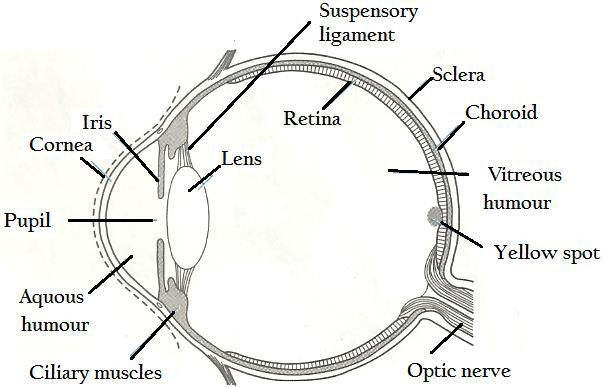​​ Parts of the human Eye ​​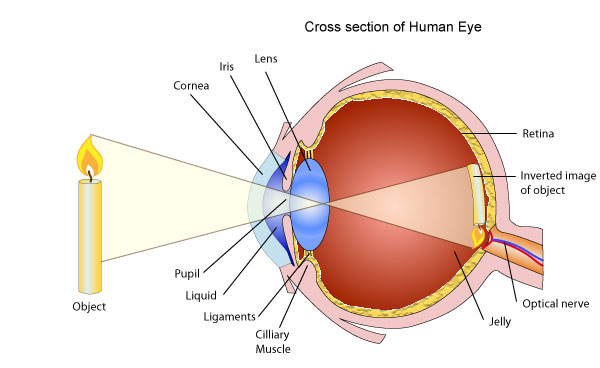##### Cornea​​​​

The cornea is the transparent, outer part of the eye. It is the primary focusing tool of the eye. The outer layer of the cornea is known as the​​ epithelium.​​

Function of cornea:  ​​ ​​​​ Its main job is to protect the eye​​

##### Iris

The iris is the part of the eye which is responsible for one’s eye colour. ​​

Function of Iris:  ​​​​ It acts like diaphragm of the camera, dilating and constricting the pupil to allow more or less light into the eye.  ​​​​

##### Pupil​​ ​​

The pupil is the dark opening in the centre of the colored iris that controls the amount of light that enters the eye. ​​

The pupil functions in the same way as the aperture of a camera. ​​

The size of the pupil determines the amount of light entering the eye. ​​

##### Lens.​​

The lens is the part of the eye immediately behind the iris. ​​

Function of lens: ​​ To focus light rays on the retina.​​

In persons under 40 years of age, the lens is soft and flexible, allowing for fine focusing from a wide variety of distance​​ ​​

##### Retina​​ ​​

The retina is the membrane lining the back of the eye that contains photoreceptor cells it reacts to the presence and intensity of light by sending an impulse to the brain via the optic nerve.​​

The retina compares to the film in a lens camera. ​​

##### Optic nerve​​ ​​

The optic nerve (million nerve fibres) is the structure which takes the information from the retina as electrical signals to the brain ​​

##### Sclera​​ ​​

The sclera is the white, tough wall of the eye. Its function is to protects the eye​​

##### Vitreous humour​​ ​​

The vitreous humour is a jelly-like substance that fills the body of the eye. It is normally clear ​​

The Function of vitreous humour ​​ ​​

To maintain the eye pressure. ​​

It also helps in focusing light rays ​​

Accommodation​​ ​​

Accommodation is the process whereby an eye focal length adjusted to see distant objects ​​

##### ​Eye Defects​​ ​​

Eye defect is the phenomenon where by eye defeat to see clearly. ​​

There are two common eye defects.​​

• Myopia (short-sightedness defect) ​​
• Hypermetropia ​​ (long-sightedness defect) ​​
##### ​​ Myopia​​ ​​

Occur when a person can see near objects clearly but cannot see distant objects clearly.​​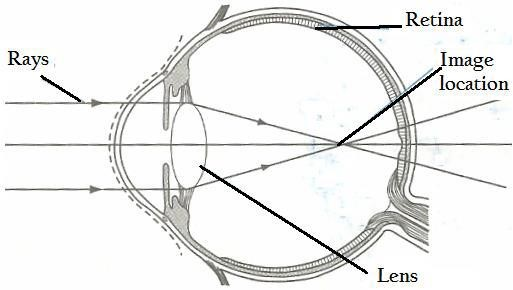​​ Causes​​ ​​

When the eyeball is too long ​​

When the refractive power of the lens is too strong​​

##### ​​Correction​​ ​​

To wear suitable concave lenses to diverge the rays from distant objects before they reach the eye. ​​ Diagram: ​​

#####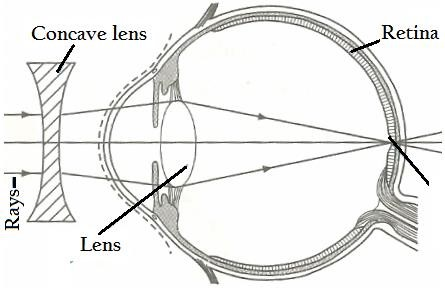​​Hypermetropia (Long – sightedness )  ​​  ​​ ​​

Occur when a person cannot see near objects clearly but can see distant objects clearly.​​

#####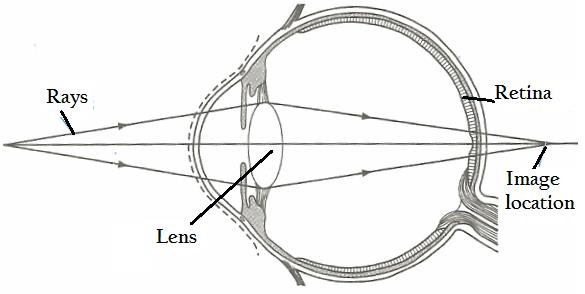Causes​​ ​​

When the eye ball is too short​​

When ciliary muscles are weak such that unable to change the shape of the eye lens in order to focus the image (occurs when the refractive power of the eye lens is too​​ weak)​​

##### ​Correction ​​

The defect can be corrected by wearing suitable convex lenses so that the rays from the near object are made to converge and focus on the retina. See the fig below ​​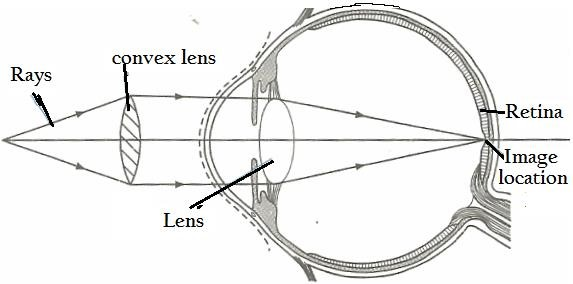​​

##### ​Other eye defects can be categorized into:-​​

Astigmatism​​

Occurs when the image is distorted because light rays are blocked from meeting at a common focus.​​

Causes ​​ when the cornea shape is like an oblong rugby ball and not spherical in shape as normal​​

correction: ​​

Can be corrected by using cylindrical lenses​​

Presbyopia  ​​​​

Occurs when the centre of the eye lens hardens making it unable to ​​ accommodate near vision​​ ​​

correction: ​​

Eye glasses with progressive lenses are prescribed to correct or improve the condition ​​

##### ​Similarities of Human Eye and Lens Camera​​ ​​
• Both have a convex lens system to focus the image ​​
• Both save images​​
• Both form a real, reduced and inverted image ​​
• The amount of light entering is controlled by a variable aperture ​​
• They both have surfaces on which the image is formed ​​
• The retina behaves like the photographic screen of a camera​​
##### Eye ​​

Lens is hard glass​​

Lens is soft and elastic​​

Thickness of lens does not change​​

Thickness of lens changes​​

Image is focused by moving the lens​​

Image is focused by changing the thickness of the lens​​

Only the lens refracts the light ​​

Aqueous and vitreous humour refracts the light​​

Diaphragm can be altered ​​

Iris alters itself​​

Cameras do not have blind spot​​

Human eyes have blind spot​​

​​Example​​

1. The far point of a myopic person is 40 cm. What should be the power of the lens that he must use to see clearly?​​

Soln:​​

Given: ​​ f = 40 cm = 0.4 m, Power =?​​

#### ​From:  ​​ ​​ ​​ ​​​​​​Class Activity – 5​​

If the focal distance of the converging lens is 5 cm the object distance is 4 cm. Find the magnification of the image ​​ ​​(ANS: ​​ M = ​​ 6 cm)​​

Given that the focal length of the simple microscope is 12cm.Find the magnification of the image of the object distances. ​​ ​​​​ (ANS: ​​ M = 8.1)​​

Given that an object 2m high is placed 2010cm in front of the lens camera of focal length 10cm.calculate the minimum size of the film frame. ​​ ​​ ​​ ​​ ​​ ​​​​ (ANS:  ​​​​ M = 0.0005)​​

The lantern projector uses a slide of 2 cm by 2 cm, 2×2 to produce a picture 1 m by 1m on a screen 12cm from the projection lens. How far from the lens must the slide be?  ​​​​

A telescope is consisting of two converging lens of lens at focal length 25 and 4 respectively. The final image is found at distinct vision that is 25 cm in front of the eyepiece lens. Find the position of the first image from the eyepiece.​​ ANS: Ue ​​​​ = 3.4cm​​

An astronomer telescope has its 2 lens 78 cm apart. If the objective lens has a focal length of 75.5 cm, what is the magnification produced by the telescope under normal vision ​​ ​​ ANS: ​​ The magnification is 30.2​​

A compound microscope has an objective lens of focal length 2 cm and eye piece lens of focal length 6cm.An object is placed 2.4 cm from an objective lens. If the distance between the objective lens and eye piece lens is 19 cm. Find  ​​ ​​ ​​ ​​ ​​ ​​ ​​ ​​ ​​ ​​ ​​ ​​ ​​ ​​ ​​ ​​ ​​ ​​ ​​ ​​ ​​ ​​ ​​ ​​ ​​ ​​ ​​ ​​ ​​ ​​ ​​ ​​ ​​ ​​ ​​ ​​ ​​​​ (a) ​​ The distance of the final image from the eyepiece lens  ​​ ​​ ​​ ​​ ​​ ​​ ​​ ​​ ​​ ​​ ​​ ​​ ​​ ​​ ​​ ​​ ​​ ​​ ​​ ​​ ​​ ​​ ​​ ​​ ​​ ​​ ​​ ​​ ​​ ​​ ​​ ​​ ​​ ​​ ​​ ​​ ​​ ​​ ​​ ​​ ​​ ​​ ​​(b) Compound magnification​​ ANS: ​​ The final image is at infinity and M = ∞​​

A certain microscope consists of 2 converging lenses of focal length 4 cm and 10 cm for objective 3 eyepiece lenses respectively. The 2 lenses are separated by the distance of 30cm.The instrument is focused so that the image is at infinity. Calculate the position of the object and the magnification of the objective lens.  ​​ ​​ ​​ ​​​​

(ANS: ​​ The magnification produced by the eye piece lens is 4)​​

A simple microscope has a focal length of 15 cm ​​

(a)What is the maximum magnification of the lens (ANS: 2.667)​​

(b)What is the magnification of this lens when the eye is relaxed  ​​ ​​ ​​ ​​​​ (ANS: 1.667)​​

The near point of a longsighted patient is 90 cm ​​. Determine the focal length of a lens that can be used to enable the patient ​​ clearly see objects that are 25 cm from the eye ​​

(a) What is the power of the lens ​​

(b) What is the magnification of the lens?​​

A short sighted person is unable to clearly see objects that are beyond 150 cm from the eye .Determine the focal length ,power and magnification of the lens that should be used to detect the eye defect​​

A patient requires a lens of ​​ -5 diopters in order to see far away objects​​ clearly (a) Determine the Focal length of the lens used  (b) Far point of the patient ‘s eye​​

Define the term ‘’accommodation’’ as used in the human eye ​. Give two similarities and 2 differences between the human eye and the camera ​​

State one advantage of human eye over a lens camera​​

The near point of an eye is 50 cm ​​. What focal length lens should be used so that the eye can clearly see an  ​​ ​​​​ object 25 cm away?  ​​​​ (ANS: f = 50 cm)​​

What is the power of this lens? ​​ (ANS: 2 diopters)​​

(a) State the causes of the short – sightedness and long – sightedness  ​​ ​​ ​​ ​​ ​​ ​​ ​​ ​​ ​​ ​​ ​​ ​​ ​​ ​​ ​​ ​​ ​​ ​​ ​​ ​​ ​​ ​​ ​​ ​​ ​​ ​​ ​​ (b) Use a ray diagram to show how ​​

(i) Short – sightedness in a human eye can be corrected  ​​​​

(ii) Long – sightedness can be corrected  ​​ ​​ ​​ ​​ ​​ ​​ ​​ ​​ ​​ ​​ ​​ ​​ ​​ ​​ ​​ ​​ ​​ ​​ ​​ ​​ ​​ ​​ ​​ ​​ ​​ ​​ ​​ ​​ ​​ ​​ ​​ ​​ ​​ ​​ ​​ ​​ ​​ ​​ ​​ ​​ ​​ ​​ ​​ ​​ ​​ ​​ ​​ ​​ ​​ ​​ ​​ ​​ ​​ ​​ ​​ ​​ ​​ ​​ ​​ ​​ ​​ ​​ ​​ ​​ ​​ ​​​​

A farsighted man has a near point of 100 cm .Wearing his glasses, he can see objects that are 25 cm away .What is the focal length of the lens in his glasses?​​

In a compound microscope ,the focal length of the objective lens is 4.0 cm and that of the eyepiece is 3.3 ​​ cm and they are placed at a distance of 15.0 cm .A real object of size 2 mm is placed 6 cm from the objective lens .By using the lens ​​ formula , Calculate ​​

(a) Position of the final image (ANS: V = 12 cm) ​​ ​​ ​​ ​​ ​​ ​​ ​​ ​​ ​​ ​​ ​​​​ (b) The size of the final image viewed by the eye (ANS: VFINAL IMAGE​​ = 33 cm) ​​ ​​ ​​ ​​ ​​ ​​ ​​ ​​  (c) Magnification produced by the arrangement of the lenses (ANS: HI​​ = 44 mm) ​​ ​​ ​​ ​​ ​​ ​​ ​​ ​​ ​​ ​​ ​​ ​​ ​​​​

(a) Differentiate between a simple microscope and a compound microscope ​​

(b) With the aid of a diagram describe how a compound microscope works. ​​ A compound microscope has an objective lens of focal length 2cm and an eyepiece of focal length 6 cm. An object is placed 2.4 cm from the objective lens​​

.If the distance between the objective lens and the eyepiece is 17m ,find ​​

(i) The distance of the final image from the eyepiece  ​​ ​​ ​​ ​​ ​​ ​​ ​​ ​​ ​​ ​​ ​​ ​​ ​​ ​​ ​​ ​​ ​​ ​​ ​​ ​​ ​​ ​​ ​​ ​​ ​​ ​​ ​​ ​​ ​​ ​​ ​​ ​​ ​​ ​​ ​​ ​​ ​​ ​​ ​​ ​​ ​​ ​​ ​​ ​​ ​​ ​​ ​​ ​​ ​​ ​​ ​​ ​​ ​​(ii) The linear magnification​​

Small object is placed ​​ 3cm from the lens of a simple microscope .If the focal length of the lens is 5 cm ,Find the linear magnification produced by the simple microscope .How far from the lens would you place the object in order to obtain maximum magnification of the image ?​​

A compound microscope expected to have a magnification of X600 has a tube length of 12 cm and the focal length of the objective lens is 0.5 cm Determine the expected focal length of the eye piece​​

A magnifying glass of focal length of 15 cm is used to view an object so as to obtain maximum magnification ​​

• Where should the object be placed?​​
• What is the magnification of the magnifying lens?​​

A compound microscope ​​ has an objective lens of focal length 25 cm and an eyepiece with focal length 14 cm, If it has a tube of length 36 cm determine the magnification of the microscope ​​

A lens of focal lens 10 cm is positioned from an object so as to obtain maximum magnification of the image .Determine the:​​

(A) Object distance  ​​ ​​ ​​ ​​ ​​ ​​ ​​ ​​​​ (b) Image distance  ​​ ​​ ​​ ​​ ​​ ​​ ​​ ​​ ​​ ​​​​ (c ) Magnification ​​

A lens camera is to be used to take a picture of a man 2 m tall .If the lens of the camera has a focal length of 10 cm , Calculate the minimum size of the film frame required ,given that the man is 20.1 m from the camera​​

A certain microscope consists of two converging lenses of focal lengths 10 cm and 4 cm for the objective and eye piece respectively .The two lenses are separated by a distance of 30 cm .The instrument is focused so that the final image is at infinity .Calculate the position of the and the magnification of the objective lens ​​ (ANS u = 16.25 cm, m = 1.6)​​

Draw a clearly labeled diagram of a lens camera and explain briefly how the image of an object is focused on the film .A camera with a lens of focal length 15 cm is used to take a photograph of a man standing 4.5 m from the lens .Find the length of the image formed if the man is 1.75 m tall​​

Describe with the aid of a diagram the optical system of the projection lantern .A projection lantern is to be used for the projection of slides measuring 7.5 cm by 7.5 cm onto a screen measuring 2.1 m by 2.1 m .If the distance between the projection lens and the screen is 6 m, Find the focal length of the lens ​​

Describe how would you construct an astronomical telescope. What will be the maximum distance between the objective lens and the eyepiece lens?. Draw a ray diagram to illustrate the paths of two rays from a point on an object which is not on the axis of the telescope​​

Define and explain the terms near point and far point as applied to the human eye .Draw diagrams to illustrate the defects of long and short sight .How can each defect be corrected by using lenses ?​​

A man whose least distance of distinct vision of 75 cm wants spectacles to allow him to read a book held at a distance of 25 cm from his eyes. Find the focal length of the lens he needs .Discuss briefly whether a short – sighted person can use a telescope without wearing any spectacles ​​

Draw a clearly labelled diagram of the human eye and explain how it can focus near and distant objects. How does the human eye resemble the lens camera?​​

A​​ telescope of 5.0 m diameter reflector of focal length 18.0 m is used to focus the image of the sun. Using the distance of the sun from the earth and diameter of the sun as 1.5 x 1011​​ m and 1.4 x 109​​ m respectively, calculate the:​​

Position of the image of the sun ( v = f =18 cm, since the object is at infinity)​​

Diameter of the image of the sun(image size)​​ A​​ person whose sight is normal wishes to view objects which are 5 cm from his eyes. Find the focal length of the lenses needed for his spectacles ​​

When is a person said to be suffering from long sight? Draw a diagram of the eye to show how this defect may be corrected by the use of a suitable type of lens.​​

Mention two ways in which a photographic camera is similar to the human eye and one way in which it is different​​

A​​ converging lens has a focal length of 5 cm (ANS: P = 20 D) (i)​​ What is the power of the lens?​​ (ii) If this lens were used in an astronomical refracting telescope, for which part of the telescope would it be most suitable? ​​ (iii)What would be the distance between the two lenses if the telescope were in normal adjustment (i.e with the final image at infinity)?​​ (ANS 🙁𝒍 = 𝒇𝒐​​ + 𝒇𝒆) →

𝒔𝒖𝒎 𝒐𝒇 𝒇𝒐𝒄𝒂𝒍 𝒍𝒆𝒏𝒈𝒕𝒉𝒔)​​

Explain the terms real image and virtual image​​

Describe the construction of a photographic camera. If the focal length of the camera lens is 20 cm, how far away from the film must the lens be set in order to photograph an object 100 cm from the lens? (ANS: v = 25 cm)​​ ​​

A slide projector using a slide 5 cm x 5 cm produces a picture 3 m x 3 m on a screen placed at a distance of 24 m from the projection lens. How far from the lens must the slide be? (ANS: 40 cm x 40 cm)​​ ​​